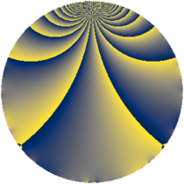# Properties

 Label 637.2.kLevel $637$ Weight $2$ Character orbit 637.k Rep. character $\chi_{637}(459,\cdot)$ Character field $\Q(\zeta_{6})$ Dimension $86$ Newform subspaces $10$ Sturm bound $130$ Trace bound $5$

# Related objects

## Defining parameters

 Level: $$N$$ $$=$$ $$637 = 7^{2} \cdot 13$$ Weight: $$k$$ $$=$$ $$2$$ Character orbit: $$[\chi]$$ $$=$$ 637.k (of order $$6$$ and degree $$2$$) Character conductor: $$\operatorname{cond}(\chi)$$ $$=$$ $$91$$ Character field: $$\Q(\zeta_{6})$$ Newform subspaces: $$10$$ Sturm bound: $$130$$ Trace bound: $$5$$ Distinguishing $$T_p$$: $$2$$, $$3$$

## Dimensions

The following table gives the dimensions of various subspaces of $$M_{2}(637, [\chi])$$.

Total New Old
Modular forms 146 102 44
Cusp forms 114 86 28
Eisenstein series 32 16 16

## Trace form

 $$86 q + 2 q^{3} - 78 q^{4} + 6 q^{6} - 41 q^{9} + O(q^{10})$$ $$86 q + 2 q^{3} - 78 q^{4} + 6 q^{6} - 41 q^{9} - 9 q^{10} - 3 q^{11} + 2 q^{12} + 4 q^{13} - 15 q^{15} + 58 q^{16} + 22 q^{17} + 51 q^{18} - 6 q^{19} + 6 q^{20} + 10 q^{23} - 18 q^{24} + 35 q^{25} - 6 q^{26} - 22 q^{27} - 2 q^{29} + 26 q^{30} - 15 q^{31} + 15 q^{33} + 37 q^{36} - 16 q^{38} - 21 q^{39} + 4 q^{40} + 15 q^{41} - 22 q^{43} - 48 q^{44} - 14 q^{48} + 30 q^{50} - 36 q^{51} + 5 q^{52} + 17 q^{53} + 24 q^{55} + 111 q^{58} + 129 q^{60} + 2 q^{61} - 38 q^{62} - 16 q^{64} - 70 q^{65} + 43 q^{66} - 54 q^{67} - 10 q^{68} - 7 q^{69} - 75 q^{71} - 159 q^{72} - 57 q^{73} - 6 q^{74} + 6 q^{75} + 42 q^{76} - 123 q^{78} + 6 q^{79} + 78 q^{80} - 23 q^{81} + 4 q^{82} - 9 q^{85} + 114 q^{86} + 26 q^{87} + 29 q^{88} + 12 q^{90} + 62 q^{92} + 14 q^{94} - 70 q^{95} - 12 q^{96} + 12 q^{97} + O(q^{100})$$

## Decomposition of $$S_{2}^{\mathrm{new}}(637, [\chi])$$ into newform subspaces

Label Dim $A$ Field CM Traces $q$-expansion
$a_{2}$ $a_{3}$ $a_{5}$ $a_{7}$
637.2.k.a $2$ $5.086$ $$\Q(\sqrt{-3})$$ None $$0$$ $$-2$$ $$-3$$ $$0$$ $$q+(-1+2\zeta_{6})q^{2}-2\zeta_{6}q^{3}-q^{4}+(-2+\cdots)q^{5}+\cdots$$
637.2.k.b $2$ $5.086$ $$\Q(\sqrt{-3})$$ None $$0$$ $$-1$$ $$-3$$ $$0$$ $$q+(1-2\zeta_{6})q^{2}-\zeta_{6}q^{3}-q^{4}+(-2+\cdots)q^{5}+\cdots$$
637.2.k.c $2$ $5.086$ $$\Q(\sqrt{-3})$$ None $$0$$ $$2$$ $$3$$ $$0$$ $$q+(-1+2\zeta_{6})q^{2}+2\zeta_{6}q^{3}-q^{4}+(2+\cdots)q^{5}+\cdots$$
637.2.k.d $4$ $5.086$ $$\Q(\sqrt{-3}, \sqrt{-7})$$ None $$0$$ $$-1$$ $$-3$$ $$0$$ $$q+(\beta _{1}-\beta _{3})q^{2}+(1-2\beta _{1}-2\beta _{2}+\beta _{3})q^{3}+\cdots$$
637.2.k.e $4$ $5.086$ $$\Q(\sqrt{-3}, \sqrt{-13})$$ None $$0$$ $$0$$ $$0$$ $$0$$ $$q+(1-2\beta _{2})q^{2}-q^{4}+\beta _{1}q^{5}+(1-2\beta _{2}+\cdots)q^{8}+\cdots$$
637.2.k.f $4$ $5.086$ $$\Q(\sqrt{-3}, \sqrt{-7})$$ None $$0$$ $$1$$ $$3$$ $$0$$ $$q+(\beta _{1}-\beta _{3})q^{2}+(-1+2\beta _{1}+2\beta _{2}+\cdots)q^{3}+\cdots$$
637.2.k.g $12$ $5.086$ 12.0.$$\cdots$$.1 None $$0$$ $$0$$ $$-6$$ $$0$$ $$q+(-\beta _{4}+\beta _{8})q^{2}+(\beta _{2}+\beta _{9})q^{3}+(-1+\cdots)q^{4}+\cdots$$
637.2.k.h $12$ $5.086$ 12.0.$$\cdots$$.1 None $$0$$ $$0$$ $$6$$ $$0$$ $$q+(-\beta _{4}+\beta _{8})q^{2}+(-\beta _{2}-\beta _{9})q^{3}+\cdots$$
637.2.k.i $12$ $5.086$ 12.0.$$\cdots$$.1 None $$0$$ $$3$$ $$3$$ $$0$$ $$q+\beta _{9}q^{2}+(-\beta _{1}-\beta _{4}-\beta _{6})q^{3}+(-1+\cdots)q^{4}+\cdots$$
637.2.k.j $32$ $5.086$ None $$0$$ $$0$$ $$0$$ $$0$$

## Decomposition of $$S_{2}^{\mathrm{old}}(637, [\chi])$$ into lower level spaces

$$S_{2}^{\mathrm{old}}(637, [\chi]) \cong$$ $$S_{2}^{\mathrm{new}}(91, [\chi])$$$$^{\oplus 2}$$